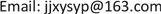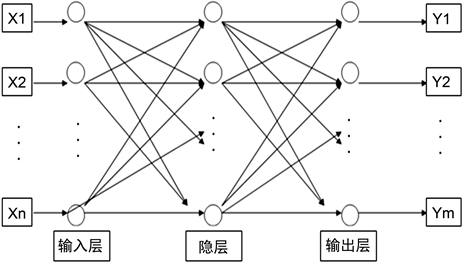﻿ 组合预测模型的有效性分析——以中国人口老龄化预测为例 The Effectiveness Analysis of Combined Forecasting Model—Based on Forecast of China’s Aging of Population

Statistics and Application
Vol. 09  No. 01 ( 2020 ), Article ID: 33946 , 8 pages
10.12677/SA.2020.91005

The Effectiveness Analysis of Combined Forecasting Model

—Based on Forecast of China’s Aging of Population

Yanpeng Sun

School of Economics, Qingdao University, Qingdao ShandongReceived: Dec. 23rd, 2019; accepted: Jan. 7th, 2020; published: Jan. 14th, 2020ABSTRACT

According to the modeling theory of quadratic exponential smoothing forecasting model, the modified grey forecasting model and BP neural network forecasting model, combined forecasting model of the aging of population is constructed, and it is concluded that both within and outside the sample forecast, combined forecasting model has better forecasting effect than single models. And then it uses combined forecasting model to predict the level of the aging of population in our country from 2017 to 2022, the forecasting results show that the future of aging problem in our country is increasingly serious. Therefore, it puts forward four suggestions: First, appropriately improve the birth rate; second, vigorously develop the aging industry; third, promote the process of urbanization; fourth, deepen the reform of urban and rural pension systems.

Keywords:The Aging of Population, Combined Forecasting Model, Quadratic Exponential Smoothing Prediction, Modified Grey Prediction, BP Neural Network Prediction——以中国人口老龄化预测为例1. 引言

2. 模型简介

2.1. 二次指数平滑预测法

${\stackrel{^}{X}}_{t+T}={a}_{t}+{b}_{t}T$ (1)

2.2. 修正的灰色预测法

${\stackrel{^}{X}}^{\left(1\right)}\left(k+1\right)=\left[{X}^{\left(0\right)}\left(1\right)-\frac{b}{a}\right]{\text{e}}^{-ak}+\frac{b}{a}$ (2)

${\stackrel{^}{X}}^{\left(0\right)}\left(k+1\right)=\left(1-{\text{e}}^{a}\right)\left[{X}^{\left(0\right)}\left(1\right)-\frac{b}{a}\right]{\text{e}}^{-ak}$ (3)

${\stackrel{^}{E}}^{\left(1\right)}\left(k+1\right)=\left[{E}^{\left(0\right)}\left(1\right)-\frac{{b}_{E}}{{a}_{E}}\right]{\text{e}}^{-{a}_{E}k}+\frac{{b}_{E}}{{a}_{E}}$ (4)

${\stackrel{^}{X}}^{\left(0\right)}\left(k+1\right)=\left(1-{\text{e}}^{a}\right)\left[{X}^{\left(0\right)}\left(1\right)-\frac{b}{a}\right]{\text{e}}^{-ak}+\delta \left(k-{k}_{0}\right)\left(1-{\text{e}}^{{a}_{E}}\right)\left[{E}^{\left(0\right)}\left(1\right)-\frac{{b}_{E}}{{a}_{E}}\right]{\text{e}}^{-{a}_{E}k}$ (5)

2.3. BP神经网络预测法

BP神经网络是一种单向传播的多层前向网络 ，本文选用较为典型的三层结构网络(即包括输入层、隐层和输出层)进行预测，其结构如图1所示。Figure 1. BP neural network topology

2.4. 组合预测模型

1969年，Bates和Granger开创了组合预测模型方法，是根据一定的准则赋予各个单项模型一定的权重，而后根据权重组合到一起形成的新模型 。该模型权重的设定方法主要有：等权法、简单加权平均法、误差平方和倒数法等，由于前两种方法较为简单，但存在较大的误差，因此本文采用误差平方和倒数法来设定权重。则第i个单项模型的权重可表示为：

${w}_{i}={D}_{i}^{-1}/{\sum }_{i=1}^{n}{D}_{i}^{-1}$ (6)

${D}_{i}={\sum }_{t=1}^{T}{\left({x}_{t}-{\stackrel{^}{x}}_{it}\right)}^{2}$ (7)

${\stackrel{^}{x}}_{t}={w}_{1}{\stackrel{^}{x}}_{1t}+{w}_{2}{\stackrel{^}{x}}_{2t}+{w}_{3}{\stackrel{^}{x}}_{3t}$ (8)

3. 组合预测模型预测的有效性分析

3.1. 组合预测模型的构建

3.1.1 数据来源

3.1.2. 构建单项预测模型

1) 二次指数平滑预测Table 1. The sums of squared error within the sample forecast by the different values of a

2) 修正的灰色预测

$k<5$ 时， ${\stackrel{^}{X}}^{\left(0\right)}\left(k+1\right)=4.1191{\text{e}}^{0.0238k}$

$k\ge 5$ 时， ${\stackrel{^}{X}}^{\left(0\right)}\left(k+1\right)=4.1191{\text{e}}^{0.0238k}+0.9030{\text{e}}^{0.0075k}-1.0402$

3) BP神经网络预测

3.1.3. 构建组合预测模型

${\stackrel{^}{x}}_{t}=0.2773{\stackrel{^}{x}}_{1t}+0.2946{\stackrel{^}{x}}_{2t}+0.4281{\stackrel{^}{x}}_{3t}$ (9)

3.2. 预测的有效性分析

1) 预测有效性的评价指标选取

$\text{MSFE}=1/T{\sum }_{t=1}^{T}{\left({x}_{t}-{\stackrel{^}{x}}_{t}\right)}^{2}$ (10)

2) 样本内预测的有效性分析Table 2. The comparison of MSFE within the sample forecast in four forecasting model

3) 样本外预测的有效性分析Table 3. The comparison of MSFE outside the sample forecast in four forecasting model

4. 我国人口老龄化预测

${\stackrel{^}{x}}_{t}=0.2952{\stackrel{^}{x}}_{1t}+0.3239{\stackrel{^}{x}}_{2t}+0.3809{\stackrel{^}{x}}_{3t}$ (11)Table 4. Foercast of China’s aging of population from 2017 to 2022

5. 结论与政策建议

The Effectiveness Analysis of Combined Forecasting Model—Based on Forecast of China’s Aging of Population[J]. 统计学与应用, 2020, 09(01): 39-46. https://doi.org/10.12677/SA.2020.91005

1. 1. United Nations (2015) World Population Prospects: The 2015 Revision. United Nations, New York.

2. 2. Siliverstovs, B., Kholodilin, K.A. and Thiessen, U. (2011) Does Aging Influence Structural Change? Evidence from Panel Data. Economic Systems, 35, 244-260. https://doi.org/10.1016/j.ecosys.2010.05.004

3. 3. Ansah, J.P., Eberlein, R.L., Love, S.R., et al. (2014) Implications of Long-Term Care Capacity Response Policies for an Aging Population: A Simulation Analysis. Health Policy, 116, 105-113. https://doi.org/10.1016/j.healthpol.2014.01.006

4. 4. 翟振武, 郑睿臻. 人口老龄化与宏观经济关系的探讨[J]. 人口研究, 2016, 40(2): 75-87.

5. 5. 李杏, 章孺, M.W. Luke Chan. 人口老龄化对产业结构的影响——基于SYS-GMM的分析[J]. 河海大学学报(哲学社会科学版), 2017, 19(1): 29-36+89.

6. 6. 张振华. 基于灰色GM(1, 1)模型的城市人口老龄化预测[J]. 统计与决策, 2015(19): 76-79.

7. 7. 张荣艳, 马艳琴, 郝淑双. 残差灰色预测模型在我国老龄人口预测中的应用[J]. 数学的实践与认识, 2013, 43(16): 162-166.

8. 8. 陈毅华, 李永胜, 苏昌贵, 孙峰华. 径向基神经网络模型在人口老龄化预测中的应用——以湖南省为例[J]. 经济地理, 2012, 32(4): 32-37.

9. 9. 黄健元. 基于Leslie方程预测的江苏省人口老龄化特征分析[J]. 南京师大学报(社会科学版), 2010(3): 46-50.

10. 10. 陈光慧, 蔡远飞, 李凤. 我国人口老龄化趋势预测与结构分析——基于非参数自回归模型[J]. 西北人口, 2014, 35(4): 81-87.

11. 11. Rapach, D.E. and Strauss, J.K. (2008) Structural Breaks and Garch Models of Exchange Rate Volatility. Journal of Applied Econometrics, 23, 65-90. https://doi.org/10.1002/jae.976

12. 12. Altug, S. (2015) Forecasting Inflation Using Survey Expectations and Target Inflation: Evidence for Brazil and Turkey. International Journal of Forecasting, 32, 138-153. https://doi.org/10.1016/j.ijforecast.2015.03.010

13. 13. 宋晓华, 祖丕娥, 伊静, 刘达. 基于改进GM(1, 1)和SVM的长期电量优化组合预测模型[J]. 中南大学学报(自然科学版), 2012, 43(5): 1803-1807.

14. 14. 熊巍, 祁春节, 高瑜, 杨春. 基于组合模型的农产品市场价格短期预测研究——以红富士苹果、香蕉、橙为例[J]. 农业技术经济, 2015(6): 57-65.

15. 15. Dahiya, R.C. and Gross, A.J. (1974) Adaptive Exponential Smoothing Models for Reliability Estimation. IEEE Transactions on Reliability, R-23, 332-334. https://doi.org/10.1109/TR.1974.5215297

16. 16. 刘思峰, 杨英杰, 吴利丰. 灰色系统理论及其应用[M]. 北京: 科学出版社, 1999: 113-116.

17. 17. 杨德平, 刘喜华. 经济预测与决策技术及MATLAB实现(第二版)[M]. 北京: 机械工业出版社, 2016: 239-250.

18. 18. Bates, J.M. and Granger, C.W.J. (2001) The Combination of Forecasts. Cambridge University Press, Cambridge, 451-468.